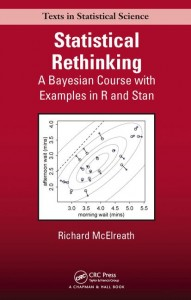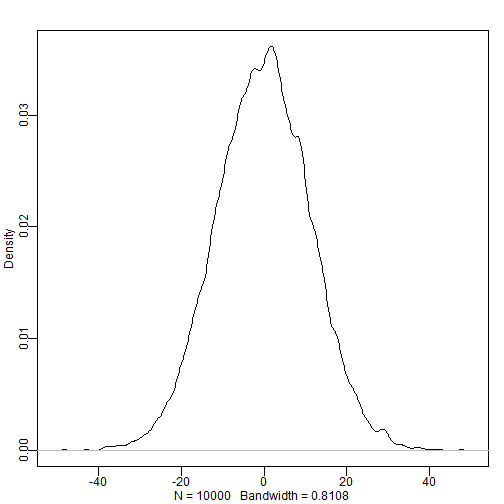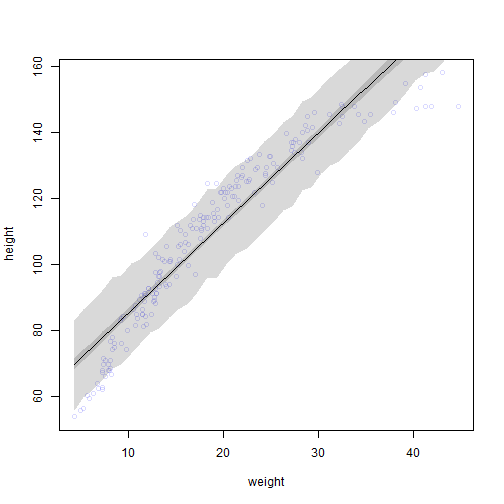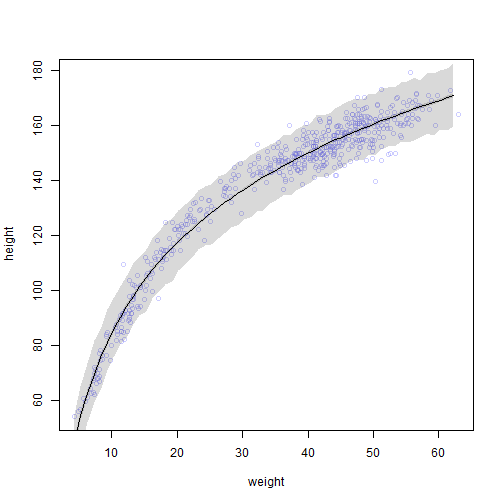ListHere I work through the practice questions in Chapter 4, “Linear Models,” of Statistical Rethinking (McElreath, 2016). I do my best to use only approaches and functions discussed so far in the book, as well as to name objects consistently with how the book does. If you find any typos or mistakes in my answers, or if you have any relevant questions, please feel free to add a comment below.

Here is the chapter summary from page 115:

This chapter introduced the simple linear regression model, a framework for estimating the association between a predictor variable and an outcome variable. The Gaussian distribution comprises the likelihood in such models, because it counts up the relative numbers of ways different combinations of means and standard deviations can produce an observation. To fit these models to data, the chapter introduced maximum a prior (MAP) estimation. It also introduced new procedures for visualizing posterior distributions and posterior predictions. The next chapter expands on these concepts by introducing regression models with more than one predictor variable.

# Setup
library(rethinking)
data(Howell1)
set.seed(4)


## Easy Difficulty

### Practice Question 4E1

In the model definition below, which line is the likelihood?

$y_i \sim \mathrm{Normal}(\mu,\sigma) \\ \mu \sim \mathrm{Normal}(0,10) \\ \sigma \sim \mathrm{Uniform}(0,10)$

Let’s label each line using the model on page 82. The first line is the likelihood, the second line is the prior for $$\mu$$, and the third line is the prior for $$\sigma$$.

Thus, the first line $$y_i \sim \mathrm{Normal}(\mu, \sigma)$$ is the likelihood.

### Practice Question 4E2

In the model definition just above, how many parameters are in the posterior distribution?

There are two parameters to be estimated in this model: $$\mu$$ and $$\sigma$$.

The $$y_i$$ is not a parameter to be estimated but rather the observed data (page 82).

### Practice Question 4E3

Using the model definition above, write down the appropriate form of Bayes’ theorem that includes the proper likelihood and priors.

Working from the example on page 83, we can insert the appropriate variables and priors to get:
$\Pr(\mu,\sigma|y) = \frac{\prod_i \mathrm{Normal} (y_i|\mu,\sigma) \mathrm{Normal} (\mu|0,10) \mathrm{Uniform}(\sigma|0,10)}{\int \int \prod_i \mathrm{Normal}(h_i|\mu,\sigma) \mathrm{Normal}(\mu|0,10) \mathrm{Uniform}(\sigma|0,10)d\mu d\sigma}$

### Practice Question 4E4

In the model definition below, which line is the linear model?

$y_i \sim \mathrm{Normal}(\mu, \sigma) \ \mu_i = \alpha + \beta x_i \ \alpha \sim \mathrm{Normal}(0, 10) \ \beta \sim \mathrm{Normal}(0, 1) \ \sigma \sim \mathrm{Uniform}(0, 10)$

Let’s label each line using the example on page 93. The first line is the likelihood, the second line is the linear model, the third line is the prior for $$\alpha$$, the fourth line is the prior for $$\beta$$, and the fifth line is the prior for $$\sigma$$.

Thus, the linear model is $$\mu_i=\alpha+\beta x_i$$.

### Practice Question 4E5

In the model definition just above, how many parameters are in the posterior distribution?

There are three parameters in the posterior distribution: $$\alpha$$, $$\beta$$, and $$\sigma$$.

The other variables are not parameters to be estimated as $$y_i$$ is the outcome variable and $$\mu$$ is now deterministic rather than probabilistic (see page 93).

## Medium Difficulty

### Practice Question 4M1

For the model definition below, simulate observed heights from the prior (not the posterior).

$y_i \sim \mathrm{Normal}(\mu, \sigma) \\ \mu \sim \mathrm{Normal}(0, 10) \\ \sigma \sim \mathrm{Uniform}(0, 10)$

To sample from the prior, we will not use the observed data but just the specified prior distributions (page 83):

sample_mu <- rnorm(1e4, 0, 10)
sample_sigma <- runif(1e4, 0, 10)
prior_y <- rnorm(1e4, sample_mu, sample_sigma)
dens(prior_y)### Practice Question 4M2

Translate the model just above into a map() formula.

To create the appropriate formula, we will use alist() and the functions beginning with “d” (page 87).

formula <- alist(
y ~ dnorm(mu, sigma),
mu ~ dnorm(0, 10),
sigma ~ dunif(0, 10)
)


### Practice Question 4M3

Translate the map() model formula below into a mathematical model definition.

flist <- alist(
y ~ dnorm(mu, sigma),
mu <- a + b*x,
a ~ dnorm(0, 50),
b ~ dunif(0, 10),
sigma ~ dunif(0, 50)
)


We just need to reverse the process shown on pages 95-96.
\begin{aligned} y_i &\sim \mathrm{Normal}(\mu, \sigma) \\ \mu_i &= \alpha + \beta x_i \\ \alpha &\sim \mathrm{Normal}(0, 50) \\ \beta &\sim \mathrm{Uniform}(0, 10) \\ \sigma &\sim \mathrm{Uniform}(0, 50) \end{aligned}

### Practice Question 4M4

A sample of students is measured for height each year for 3 years. After the third year, you want to fit a linear regression predicting height using year as a predictor. Write down the mathematical model definition for this regression, using any variable names and priors you choose. Be prepared to defend you choice of priors.

If the same sample of students are repeatedly sampled each year, then the observations are not independent and we should use a linear mixed model. However, we haven’t learned that yet in this book, so I will instead use a linear model. The question talks about “students” without specifying age, so I am going to start with a weak prior for the intercept, $$\alpha$$, that will capture likely heights for students all the way from school age children to college age young adults (from around 110 cm for a 5 year old female to around 180 cm for a 20 year old male). Similarly, I will use a weak prior for the slope, $$\beta$$, that will capture likely yearly growth rates for this wide age range (from around 7.0 cm/year for a 5 year old to around 0.5 cm/year for a 20 year old). Finally, I will use a uniform prior for the standard deviation of heights that can cover the full range if students from all ages are included.
\begin{aligned} h_{i} &\sim \mathrm{Normal}(\mu,\sigma) \\ \mu_i &= \alpha + \beta x_i \\ \alpha &\sim \mathrm{Normal}(150, 25)\\ \beta &\sim \mathrm{Normal}(4, 2)\\ \sigma &\sim \mathrm{Uniform}(0, 50) \end{aligned}

I chose a linear model without any polynomial terms or transformations because I noticed that a later question will ask for log transformation and I want an un-transformed point of comparison. For the $$alpha$$ prior, I chose a normal distribution centered on 150 cm with an SD of 25 cm; 150 cm is in the middle of the expected distribution if both school and college students are included and 25 cm is enough variability that two SDs around the mean (i.e., 100 cm to 200 cm) should include most students at the high and low end of the age distribution. For the $$beta$$ prior, I chose a normal distribution centered on 4 cm/year with an SD of 2 cm/year; 4 cm/year is in the middle of the expected distribution if both school and college students are included and 2 cm/year is enough variability that two SDs around the mean (i.e., 0 cm/year to 8 cm/year) should include most students at the high and low end of the age distribution. This also captures prior knowledge that students should only very rarely be growing less tall over time. Finally, for the $$sigma$$ prior, I chose a uniform distribution from 0 cm to 50 cm; this range includes both a tight distribution of students around the same age/height and a wide range of students at both school and college ages/heights; 50 cm is a bit high, but I want a conservative prior to begin with.

### Practice Question 4M5

Now suppose I tell you that the average height in the first year was 120 cm and that every student got taller each year. Does this information lead you to change your choice of priors? How?

Knowing that the average height at the first year was 120 cm and that every student got taller each year makes me more confident that we are talking about school age students (e.g., around 7 years old). Thus, I can narrow the range of my prior distributions to make heights and growth rates from older ages less plausible. My expectation for $$\sigma$$ is also much lower now too as I no longer expect a balanced mix of young and old students.
\begin{aligned} h_{i} &\sim \mathrm{Normal}(\mu,\sigma) \\ \mu_i &= \alpha + \beta x_i \\ \alpha &\sim \mathrm{Normal}(120, 10)\\ \beta &\sim \mathrm{Normal}(7, 1)\\ \sigma &\sim \mathrm{Uniform}(0, 20) \end{aligned}

I will center the $$\alpha$$ prior around 120 cm and decrease its SD to 10 cm to reflect our new knowledge about the average height. Similarly, I will recenter the $$\beta$$ prior around 7 cm/year and decrease its SD to 1 cm/year as these values are more consistent with school age students. Finally, I will reduce the maximum value in the $$\sigma$$ prior to 20 cm, as a higher SD is less likely with such a low average height.

### Practice Question 4M6

Now suppose I tell you that the variance among heights for students of the same age is never more than 64 cm. How does this lead you to revise your priors?

The variance is the square of $$\sigma$$, so if variance is never more than 64 cm, then $$\sigma$$ is never more than 8 cm. So we can adjust the maximum of the $$\sigma$$ prior. This information about $$\sigma$$ may also have implications for the $$\alpha$$ prior, but I am not confident enough about this relationship to update that prior.
\begin{aligned} h_{i} &\sim \mathrm{Normal}(\mu,\sigma) \\ \mu_i &= \alpha + \beta x_i \\ \alpha &\sim \mathrm{Normal}(120, 10)\\ \beta &\sim \mathrm{Normal}(7, 1)\\ \sigma &\sim \mathrm{Uniform}(0, 8) \end{aligned}

## Hard Difficulty

### Practice Question 4H1

The weights listed below were recorded in the !Kung census, but heights were not recorded for these individuals. Provide predicted heights and 89% intervals (either HPDI or PI) for each of these individuals. That is, fill in the table below, using model-based predictions.

Individual weight expected height 89% interval
1 46.95
2 43.72
3 64.78
4 32.59
5 54.63

Since we are just making predictions and not interpreting the estimates, I won’t bother centering the predictor variable. I’ll load the data, specify the map() formula and calculate the quadratic approximation (page 102).

d <- Howell1
formula <- alist(
height ~ dnorm(mu, sigma),
mu <- a + b * weight,
a ~ dnorm(140, 30),
b ~ dnorm(0, 10),
sigma ~ dunif(0, 50)
)
(m <- map(formula, data = d))

##
## Maximum a posteriori (MAP) model fit
##
## Formula:
## height ~ dnorm(mu, sigma)
## mu <- a + b * weight
## a ~ dnorm(140, 30)
## b ~ dnorm(0, 10)
## sigma ~ dunif(0, 50)
##
## MAP values:
##         a         b     sigma
## 75.515463  1.762386  9.345949
##
## Log-likelihood: -1987.71


Now we can calculate the posterior distribution of heights for each weight value in our table (page 105).

new_weight <- c(46.95, 43.72, 64.78, 32.59, 54.63)
pred_height <- link(m, data = data.frame(weight = new_weight))
expected <- apply(pred_height, 2, mean)
interval <- apply(pred_height, 2, HPDI, prob = 0.89)


Finally, we can collect the desired information in a data.frame to “complete” the table.

data.frame(
individual = 1:5,
weight = new_weight,
expected = expected,
lower = interval[1, ],
upper = interval[2, ]
)

##   individual weight expected    lower    upper
## 1          1  46.95 158.2432 157.4569 159.0932
## 2          2  43.72 152.5508 151.7869 153.2559
## 3          3  64.78 189.6659 188.4107 191.1623
## 4          4  32.59 132.9358 132.2375 133.5442
## 5          5  54.63 171.7781 170.8456 172.9093


### Practice Question 4H2

Select out all the rows in the Howell1 data with ages below 18 years of age. If you do it right, you should end up with a new data frame with 192 rows in it.

(a) Fit a linear regression to these data, using map(). Present and interpret the estimates. For every 10 units of increase in weight, how much taller does the model predict a child gets?

(b) Plot the raw data, with height on the vertical axis and weight on the horizontal axis. Superimpose the MAP regression line and 89% HPDI for the mean. Also superimpose the 89% HPDI for predicted heights.

(c) What aspects of the model fit concern you? Describe the kinds of assumptions you would change, if any, to improve the model. You don’t have to write any new code. Just explain what the model appears to be doing a bad job of, and what you hypothesize would be a better model.

First, we need to filter Howell1 to only include participants younger than 18 years old (page 96). We can check to make sure the number of row is 192 as stated in the question.

d2 <- Howell1[Howell1$age < 18, ] nrow(d2)  ##  192  Next, for (a), we need to fit a linear regression to the data using map() and then interpret the estimates given by precis(). Pages 96 and 98 work through a similar problem. # Part a formula <- alist( height ~ dnorm(mu, sigma), mu <- a + b * weight, a ~ dnorm(110, 30), b ~ dnorm(0, 10), sigma ~ dunif(0, 60) ) m <- map(formula, data = d2) precis(m, corr = TRUE)  ## Mean StdDev 5.5% 94.5% a b sigma ## a 58.35 1.40 56.11 60.58 1.00 -0.90 0.01 ## b 2.72 0.07 2.61 2.82 -0.90 1.00 -0.01 ## sigma 8.44 0.43 7.75 9.13 0.01 -0.01 1.00  The estimate of $$a$$ indicates that around 58.4 cm is a plausible height for a participant below 18 years old with a weight of 0 kg (it would have been better to center weight here, but the next part assumes you didn’t). The estimate of $$b$$ indicates that, in this sample, we can expect an increase in height of around 2.72 cm for each additional unit of weight. The estimate of $$\sigma$$ indicates that, for participants below 18 years old, the standard deviation of heights is around 8.44 cm. For each 10 unit increase in weight, the model predicts a 27.2 cm increase in height. Next, for (b), we need to plot the raw data, the MAP regression line, and the 89% HPDIs for the mean and predicted heights. These steps are described on pages 105-106. # Part b plot(height ~ weight, data = d2, col = col.alpha(rangi2, 0.3)) weight.seq <- seq(from = min(d2$weight), to = max(d2weight), by = 1) mu <- link(m, data = data.frame(weight = weight.seq)) mu.mean <- apply(mu, 2, mean) mu.HPDI <- apply(mu, 2, HPDI, prob = 0.89) lines(weight.seq, mu.mean) shade(mu.HPDI, weight.seq) sim.height <- sim(m, data = list(weight = weight.seq)) height.HPDI <- apply(sim.height, 2, HPDI, prob = 0.89) shade(height.HPDI, weight.seq)Finally, for part (c), we need to assess the model’s fit. The linear model seems to be doing a poor job predicting height at most weights. It overestimates height at both low (<10) and high (>30) weights and underestimates height for most middling (10-30) weights. The main assumption that I think are problematic here are (1) that the relationship between $$\mu$$ and weight is linear. Given what we have learned in this chapter and how the raw data appear, I might start with a polynomial (e.g., quadratic) regression. ### Practice Question 4H3 Suppose a colleague of yours, who works on allometry, glances at the practice problems just above. Your colleague exclaims, “That’s silly. Everyone knows that it’s only the logarithm of body weight that scales with height!” Let’s take your colleague’s advice and see what happens. (a) Model the relationship between height (cm) and the natural logarithm of weight (log-kg). Use the entire Howell1 data frame, all 544 rows, adults and non-adults. Fit this model, using quadratic approximation: \begin{aligned} h_i &\sim \mathrm{Normal}(\mu_i, \sigma) \\ \mu_i &= \alpha + \beta log(w_i) \\ \alpha &\sim \mathrm{Normal}(178, 100) \\ \beta &\sim \mathrm{Normal}(0, 100) \\ \sigma &\sim \mathrm{Uniform}(0, 50) \end{aligned} where $$h_i$$ is the height of individual $$i$$ and $$w_i$$ is the weight (in kg) of individual $$i$$. The function for computing a natural log in R is just log(). Can you interpret the resulting estimates? (b) Begin with this plot: plot(height ~ weight, data = Howell1), col = col.alpha(rangi2, 0.4)) Then use samples from the quadratic approximate posterior of the model in (a) to superimpose on the plot: (1) the predicted mean height as a function of weight, (2) the 97% HPDI for the mean, and (3) the 97% HPDI for predicted heights. First, for part (a), we need convert the model expressions into a MAP formula and examine its estimates. We can use the note on page 94 to see that we can simply replace weight with log(weight) in the linear model specification. d <- Howell1 formula <- alist( height ~ dnorm(mu, sigma), mu <- a + b * log(weight), a ~ dnorm(178, 100), b ~ dnorm(0, 100), sigma ~ dunif(0, 50) ) m <- map(formula, data = d) precis(m, corr = TRUE)  ## Mean StdDev 5.5% 94.5% a b sigma ## a -23.78 1.34 -25.92 -21.65 1.00 -0.99 0 ## b 47.08 0.38 46.46 47.69 -0.99 1.00 0 ## sigma 5.13 0.16 4.89 5.38 0.00 0.00 1  The estimate of $$a$$ indicates that the predicted height of an individual with a weight equal to 0 log-kg is -23.8 cm. The estimate of $$b$$ indicates that the predicted increase in height for a 1 log-kg increase in weight is 47.1 cm. The estimate of $$\sigma$$ indicates that, in the model, the standard deviation of height predictions is 5.1 cm. Next, for part (b), we need to build upon the provided plot and add to it the MAP regression line and the HPDIs for the mean and predictions as before. Page 108 provides examples similar to these tasks. plot(height ~ weight, data = d, col = col.alpha(rangi2, 0.4)) # Estimate and plot the MAP regression line and 89% HPDI for the mean weight.seq <- seq(from = min(dweight), to = max(d\$weight), by = 1)
mu <- link(m, data = data.frame(weight = weight.seq))
mu.mean <- apply(mu, 2, mean)
mu.HPDI <- apply(mu, 2, HPDI, prob = 0.97)
lines(weight.seq, mu.mean)
# Estimate and plot the 89% HPDI for the predicted heights
sim.height <- sim(m, data = list(weight = weight.seq))
height.HPDI <- apply(sim.height, 2, HPDI, prob = 0.97)Our colleague was right, this appears to be a much better fitting model.

### Session Info

sessionInfo()

## R version 3.5.1 (2018-07-02)
## Platform: x86_64-w64-mingw32/x64 (64-bit)
## Running under: Windows >= 8 x64 (build 9200)
##
## Matrix products: default
##
## locale:
##  LC_COLLATE=English_United States.1252
##  LC_CTYPE=English_United States.1252
##  LC_MONETARY=English_United States.1252
##  LC_NUMERIC=C
##  LC_TIME=English_United States.1252
##
## attached base packages:
##  parallel  stats     graphics  grDevices utils     datasets  methods
##  base
##
## other attached packages:
##  RWordPress_0.2-3     knitr_1.21           rethinking_1.59
##  usethis_1.4.0
##
## loaded via a namespace (and not attached):
##   Rcpp_1.0.0         mvtnorm_1.0-8      lattice_0.20-35
##   prettyunits_1.0.2  ps_1.3.0           assertthat_0.2.0
##   digest_0.6.18      mime_0.6           R6_2.3.0
##  plyr_1.8.4         stats4_3.5.1       evaluate_0.12
##  coda_0.19-2        highr_0.7          pillar_1.3.1
##  rlang_0.3.0.1      lazyeval_0.2.1     rstudioapi_0.8
##  callr_3.1.1        rmarkdown_1.11     stringr_1.3.1
##  loo_2.0.0          RCurl_1.95-4.11    munsell_0.5.0
##  hunspell_3.0       compiler_3.5.1     xfun_0.4
##  pkgconfig_2.0.2    base64enc_0.1-3    pkgbuild_1.0.2
##  htmltools_0.3.6    spelling_2.0       tidyselect_0.2.5
##  tibble_1.4.2       gridExtra_2.3      matrixStats_0.54.0
##  XML_3.98-1.16      crayon_1.3.4       dplyr_0.7.8
##  withr_2.1.2        MASS_7.3-50        bitops_1.0-6
##  commonmark_1.7     grid_3.5.1         jsonlite_1.6
##  gtable_0.2.0       magrittr_1.5       scales_1.0.0
##  cli_1.0.1          stringi_1.2.4      fs_1.2.6
##  bindrcpp_0.2.2     xml2_1.2.0         tools_3.5.1
##  glue_1.3.0         markdown_0.9       purrr_0.2.5
##  rsconnect_0.8.12   processx_3.2.1     yaml_2.2.0
##  inline_0.3.15      colorspace_1.3-2   bindr_0.1.1
##  XMLRPC_0.3-1


### References

McElreath, R. (2016). Statistical rethinking: A Bayesian course with examples in R and Stan. New York, NY: CRC Press.